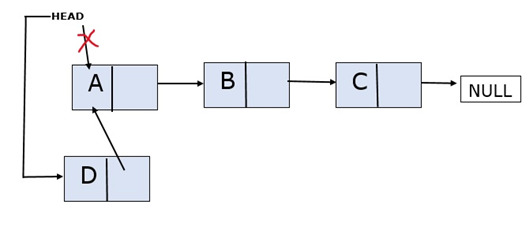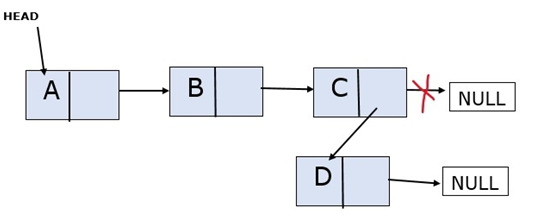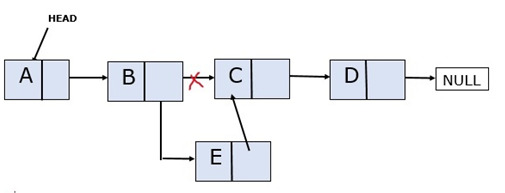## What is a Linked List

When data is not stored in continuous memory locations, it takes a lot of time to search all the memory locations to get the required data. To prevent this, we use Linked Lists.

A linked list in Python is a data structure that stores items in an ordered sequence. It consists of nodes, where each node contains the item and a reference to the next node in the list. The first node is known as the head, while the last one is referred to as tail.

Linked lists are used for efficient insertion and deletion operations since new items can be added or removed from anywhere within the list without having to move all other elements around it. Additionally, they take up less memory space than arrays since only references need to be stored instead of copies of each element.

Linked list consists of nodes. Every node contains

• Data

• Link to the next node (usually a pointer)

HEAD will contain the link to the first node. The last node will point to NULL. There are 3 cases for adding the elements into a linked list: You can add the elements at the beginning, at the end or in the middle (at any position other than the beginning and the end)

## Adding Elements At The Beginning

Consider the linked list with 3 nodes A, B and C. The new node D is to be added at the beginning.### Algorithm

Step 1 − First, create the new node that has to be added at the beginning of the linked list and allocate the data.

Step 2 − Now, Make the new node point to the head.

Step 3 − Make the head point to the address of the newly created node.

### Function Implementation

def insert_at_the_beginning(self, newVal):
newNode = Node(newVal)      #creating the new node
newNode.next = self.head    #pointing the new node to the previous first node


## Adding Elements At The End

The new node is added after the last node and the new node is pointed to NULL. Consider the linked list with 3 nodes A, B and C. The new node D is to be added at the end.### Algorithm

Step 1 − Create the new node that has to be added at the beginning of the linked list and allocate the data and make it point to NULL.

Step 2 − Traverse to the end of the linked list (link list ends when you encounter NULL)

Step 3 − Make the last node point to the newly created node

### Function Implementation

def insert_at_the_end(self,newVal):
newNode=Node(newVal)
while(temp.next):
temp=temp.next
temp.next=newNode


## Adding Elements In The Middle

The position where the new node has to be inserted will be given along with the data. We need to insert the new node at that position.

Consider the linked list with 4 nodes A,B,C and D. The new node E has to be inserted at the location 3(it means that it has added after the node B).### Algorithm

Step 1 − Create the new node that has to be added in the middle of the linked list and allocate the data.

Step 2 − Traverse the list until just before the position of the new node

Step 3 − Suppose you want to insert a new node at position ‘x’

• Traverse until x-1.

• Point the new node to (x-1.next)

• Point x-1 to the new node.

### Function Implementation

def insert_in_middle(self,newVal,pos):
newNode=Node(newVal)
for i in range(2,pos):
if temp.next!=None:
temp=temp.next
newNode.next=temp.next
temp.next=newNode


### Python Code

class Node:
def __init__(self, val):
self.val = val
self.next = None

def __init__(self):

def insert_at_the_beginning(self, newVal):
newNode = Node(newVal)      #creating the new node
newNode.next = self.head    #pointing the new node to the previous first node

def insert_at_the_end(self,newVal):
newNode=Node(newVal)
while(temp.next):
temp=temp.next
temp.next=newNode

def insert_in_middle(self,newVal,pos):
newNode=Node(newVal)
for i in range(2,pos): #for traversing to the position where you want to insert the new node
if temp.next!=None:
temp=temp.next

newNode.next=temp.next
temp.next=newNode

def Print_the_LL(self):
if(temp != None):
print("The linked list elements are:", end=" ")
while (temp != None):
print(temp.val, end=" ")
temp = temp.next
else:
print("The list is empty.")

print("\nEnter the number of elements you want to add into the linked list :")
n=int(input())
for i in range(n):
print("Chose from the following: 1.BEGINNING   2.END   3.MIDDLE")
a=int(input())

if(a==1):
print("Enter the data :  ")
data=int(input())
newList.insert_at_the_beginning(data)
elif(a==2):
print("Enter the data :  ")
data=int(input())
newList.insert_at_the_end(data)
else:
print("Enter the position: ")
pos=int(input())
print("Enter the data :  ")
data=int(input())
newList.insert_in_middle(data,pos)

newList.Print_the_LL()


### Output

Enter the number of elements you want to add into the linked list :
3
Chose from the following: 1.BEGINNING   2.END   3.MIDDLE
1
Enter the data :
112
Chose from the following: 1.BEGINNING   2.END   3.MIDDLE
2
Enter the data :
145
Chose from the following: 1.BEGINNING   2.END   3.MIDDLE
3
Enter the position:
2
Enter the data :
223
The linked list elements are: 112 223 145


Updated on: 24-Apr-2023

106 Views